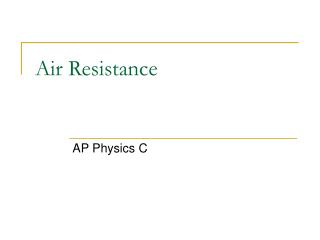DownloadDownload PresentationAir Resistance

# Air Resistance

Télécharger la présentation## Air Resistance

- - - - - - - - - - - - - - - - - - - - - - - - - - - E N D - - - - - - - - - - - - - - - - - - - - - - - - - - -
##### Presentation Transcript

1. Air Resistance AP Physics C

2. A falling object Let’s first look at the graphs of motions concerning a object that is dropped from rest. a(m/s/s) v(m/s) y(m) vT t(s) t(s) t(s) The acceleration is NOT constant but rather decreases. Here the slope decreases as TERMINAL VELOCITY, vT, is reached. With air resistance it takes MORE TIME to cover the displacement. The acceleration will decrease as the object reaches a constant speed.

3. What is the velocity function? Suppose we have an object of mass “m” that is dropped from rest in air. Assume that the air resistance function is one where it is in direct proportion with the object’s speed, FAIR=kv. What is the velocity of the object as a function of time? Note : “k” is simply a constant of proportionality) Let’s start by making a free body diagram. If the object WAS moving at terminal velocity, then the velocity would be constant and the object would be at equilibrium. kv mg

4. What is the velocity function? So then the question is, what is the velocity doing BEFORE it reaches terminal velocity? IT CHANGES! In the case we must create what is called a FIRST ORDER DIFFERENTIAL EQUATION. To do this we must simply use Newton’s second Law. kv mg

5. What is the velocity function? So what we are now looking for is a function v(t) that is subject to its initial condition. In other words, v(0)=? Start with your differential equation and isolate the differential by dividing by mass in this case. Where did this negative come from? This is the point where we could potentially mess up the math. Remember that the acceleration is DECREASING as it is moving down due to air resistance. So the CHANGE is indeed negative. Thus the negative MUST be inserted to make the equation conceptually accurate.

6. What is the velocity function? So now we must isolate the “v” term since we eventually want a velocity function. To do that we must think about which factor to pull out that still makes the statement true. Then we do what is called ISOLATE THE VARIABLES. In other words, get all your “v” like terms on one side and all your non-”v” terms or “t” terms on the other. Make sure the negative sign is on the OPPOSITE side from your “v”.

7. What is the velocity function? So now we integrate both sides using appropriate limits. On the right side we must make sure to bring out our constant before integrating. You will probably NOT need the TI-89 graphing calculator as there is an important “Calc” rule here to remember.

8. What is the velocity function? Integrating simply gives us the natural log of whatever was in denominator. Then we must APPLY the LIMITS. Also remember that Ln(a) – Ln(b) = Ln(a/b) Note: The absolute value sign was removed by looking at the term inside and deciding if it is TRUE. Since mg/k happens to be the terminal velocity we know it will be LARGER than v(t). Therefore the expression is true.

9. What is the velocity function? To get rid of the natural log we must raise both side using “e”, according to the log rule. The only thing left to do now is the algebra involved in getting v(t) by itself.

10. What is the velocity function? We have the final function but the real question is: IS IT TRUE? There is a way we can test it! Start by placing “0” in for “t”

11. Testing the velocity function When “t” is equal to 0, the velocity happens to be zero, which is what we would expect. The initial velocity is zero. We use “infinity” to express a very long time. Remember that negative sign? Remember that anything raised to the negative power is really in the denominator. Since infinity is now in the denominator, we get zero. Therefore, after a very long time the object must be moving at TERMINAL VELOCITY!